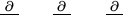# CSCI 2170 Lecture Notes - Lecture 15: Iterative Method, Surface Integral, Divergence Theorem

17 views3 pages
School
Course
ProfessorMath 241 Chapter 15 Dr. Justin O. Wyss-Gallifent
§15.1 Vector Fields
1. Deﬁne a vector ﬁeld: Assigns a vector to each point in the plane or in 3-space. Can be visualized
as loads of arrows. Can represent a force ﬁeld or ﬂuid ﬂow - both are useful.
2. Two important deﬁnitions. Often before I do these I deﬁne =
x ˆı+
y ˆ+
z ˆ
divergence and curl all make sense with how is used.
(a) The divergence ∇ · ¯
F=Mx+Ny+Pzgives the net ﬂuid ﬂow in/out of a point (very small
ball).
(b) The curl ∇ × ¯
Fgives the axis of rotation of the ﬂuid at a point.
3. For a function fwe saw the gradient fis a VF. In fact it’s a special kind of VF. Any VF which
is the gradient of a function fis conservative and the fis a potential function.
There are two facts to note:
(a) If ¯
Fis conservative then × ¯
F=¯
0 and consequently if × ¯
F6=¯
0 then ¯
Fis not conservative.
Moreover if ∇ × ¯
F=¯
0 and ¯
Fis deﬁned for all (x, y, z) then ¯
Fis conservative.
(b) If we have ¯
Fwe can tell if it’s conservative by the above method and we can ﬁnd the potential
function too using the iterative method. Make sure to do 2-variable and 3-variable cases.
§15.2 Line Integrals (of Functions and of VFs)
1. If Cis a curve and fgives the density at any point then we can deﬁne the line integral of fover/on
C, denoted RCf ds, as the total mass of C. We evaluate it by parametrizing Cas ¯r(t) on [a, b] and
then RCf ds =Rb
af(x(t), y(t), z(t))||¯r(t)|| dt. The result is independent of the parametrization
and the orientation.
Sample units: Cin cm, fin g/cm and the result in g.
2. If Cis the path of an object through a force ﬁeld ¯
Fthen we can deﬁne the line integral of ¯
F
over/on C, denoted RC¯
F·d¯r, as the total work done by ¯
Fas it traverses C. The most basic way to
evaluate it is by parametrizing Cas ¯r(t) on [a, b] and then RC¯
F·d¯r=Rb
a¯
F(x(t), y(t), z(t))·¯r(t)dt.
Some notes about line integrals of vector ﬁelds:
(a) The orientation (direction) of Cmatters. If Cis the same curve in the opposite direction
then RC¯
F·d¯r=RC¯
F·d¯r. This makes sense for work done.
(b) The parametrization in that direction doesn’t matter.
(c) There is alternate notation for this integral. We can write RCM dx +N dy +P dz which
means the same as RC(Mˆı+Nˆ+Pˆ
k)·d¯r. Watch out for things like RCM dx which looks
deceivingly like a regular integral.
Sample units: Cin cm, ¯
Fin g ·cm/s (dynes) and the result in g ·cm2/s2(ergs).
Unlock document

This preview shows page 1 of the document.
Unlock all 3 pages and 3 million more documents.